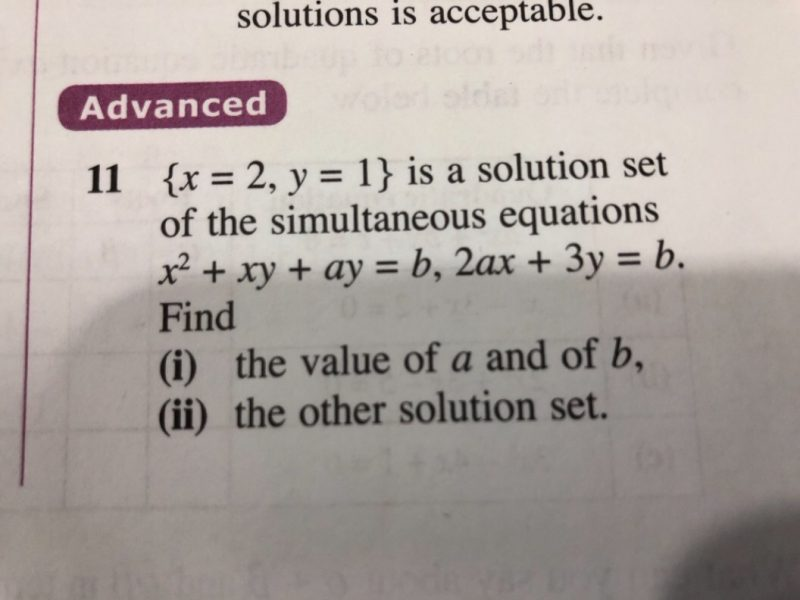# Question** Dear teacher, please help on question 11(ii). Thank you.

from i you get a=1, b=7

so x2 +xy + y =7   1)

2x + 3y = 7           2)

1) – 2)  x2 +xy -2x-2y = 0

factorize it,   x(x+y) – 2(x+y)=0

(x-2)(x+y) = 0

so x = 2(this is the solution set given in the questions) or x = -y

take x = – y and submit into eqn 2, you will get x=-7 and y =7

0 Replies 0 Likes